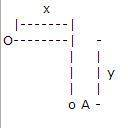# Ball rotating on axle which is rotating itself

## Homework Statement

A ball of mass m is attached via a rod of length x to an axle that rotates with angular velocity ω. You can consider the ball to be a point mass.
m = 5 kg, x = 0.3 m, y = 0.4 m, ω= 30 rad/s

(a) What is the linear momentum (direction and magnitude) of the ball?
(b) What is the angular momentum (direction and magnitude) of the ball about point A?

I've included a diagram I made.The top ball has mass m = 5 kg and the bottom ball A is rotating with angular velocity ω. Hopefully that diagram makes sense...

I = mr2
L=Iω
p=mv
v=rω

## The Attempt at a Solution

(a) p=mv
p=5kg*9m/s=45kgm/s clockwise
(b) L=Iω
L=mr2ω
This is where I am confused. It would be easy to calculate if A wasn't rotating but how do I . calculate the angular momentum of the ball about point A given the fact that A is rotating?

#### Attachments

•Mass rotating on rotating axle.JPG
2.3 KB · Views: 416

SammyS
Staff Emeritus
Homework Helper
Gold Member

## Homework Statement

A ball of mass m is attached via a rod of length x to an axle that rotates with angular velocity ω. You can consider the ball to be a point mass.
m = 5 kg, x = 0.3 m, y = 0.4 m, ω= 30 rad/s

(a) What is the linear momentum (direction and magnitude) of the ball?
(b) What is the angular momentum (direction and magnitude) of the ball about point A?

I've included a diagram I made.The top ball has mass m = 5 kg and the bottom ball A is rotating with angular velocity ω. Hopefully that diagram makes sense...

I = mr2
L=Iω
p=mv
v=rω

## The Attempt at a Solution

(a) p=mv
p=5kg*9m/s=45kgm/s clockwise
(b) L=Iω
L=mr2ω
This is where I am confused. It would be easy to calculate if A wasn't rotating but how do I . calculate the angular momentum of the ball about point A given the fact that A is rotating?
As I read this problem, there is only one ball. No ball at point A.Ah, you again. Thanks for the help! :)

I wish I could show you the diagram on my paper... it demonstrates the problem a lot better. The axle itself is rotating with angular velocity ω. There might not necessarily be a ball at the end of the axle but the point at the end of the axle is labeled A.

SammyS
Staff Emeritus
Homework Helper
Gold Member
Ah, you again. Thanks for the help! :)

I wish I could show you the diagram on my paper... it demonstrates the problem a lot better. The axle itself is rotating with angular velocity ω. There might not necessarily be a ball at the end of the axle but the point at the end of the axle is labeled A.
Do this mean you still haven't solved the problem ?

haruspex